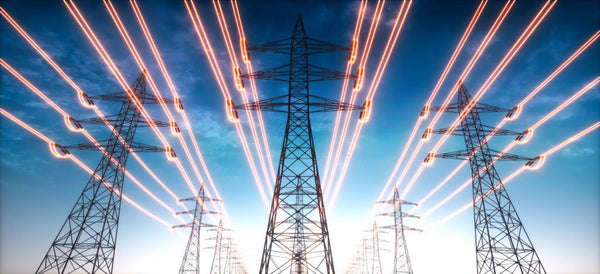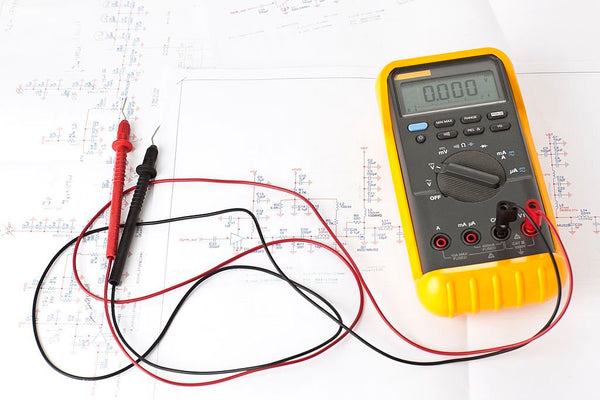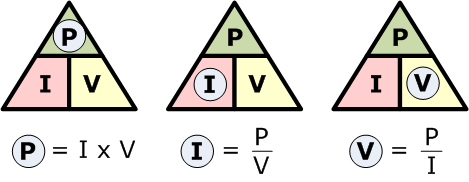Convert VA to Watts - Understanding the Units of Power - ShopSolar.com

# Convert VA to Watts

VA. Watts. Amps. Volts. These terms get thrown around in the world of solar and can easily throw us off.

However, if we take time to understand these units of power, we see that converting one of them to obtain another is easy

If you got yourself a brand new battery of generator, you will see a power rating measured in kVA.

Now, before you get frazzled and start looking for a kVA to watts calculator online, we should try to understand the unit first.

Volt amperes are made up of two units of power, multiplied together. This is of course volts and amps.

## The Different Units of Power### Volts

Voltage can be defined as the difference in electrical potential energy between two points for every unit of charge.

When we have a 9 V battery, we’re talking about the difference between the positive and negative terminals rated at 9 V.

The potential energy we’re measuring in a battery is the electromagnetic force or the repulsive and attractive forces between electrically charged particles.

For a more practical example, let’s take a smaller battery that’s measured at 3 V and connect a conductive electric wire on the positive and negative terminals.

The electrons from the negative terminal will be attached to the positive terminal at a force of 3 V.

If we remove the battery from the circuit, the electrons will flow in all directions and won’t have a flow to them. the batteries are what push these electrons around the circuit.

Understanding what volts make it easier to convert volts to watts, or volts to amps. But what are amps?

### Amps

If voltage refers to the pressure or motion of electrons in a circuit, amperes refer to the number of electrons that are flowing per second.

1 ampere is equal to 1 coulomb per second and is the base unit of electric current.

A volt-ampere (VA) is used in direct current (DC) circuits and is a measurement of electric power.

It’s used to determine how much energy a particular appliance uses and the current drawn from the circuit.

It’s achieved by multiplying volts and amps together, but we can also obtain another value by multiplying volts and amps.### Watts

In the world of solar, watts can be used to size your system or your solar needs.

Whether you’re using a household watt calculator to see how much power your home consumes, or if you’re trying to figure out the watts of an appliance, you can’t escape watts.

But what are watts?

Watts are simply a measure of power or the flow of energy. we put energy into appliances to create power. Think of humans for example.

We eat food so that we can go about our day and do work. Watts, or power, would be us doing the work.

Knowing watts, volts, and amps helps us use mAh to watts calculators to ensure we have enough power in our circuits to power our appliances.

If we don’t, our appliances could burn and smoke, rendering them broken and useless

But now that we’re able to distinguish the differences between the different units of power, what rule or law tells us that multiplying volts and amps together will give us watts?

### Watt’s Law

Watt’s law can be used to describe the relationship between 3 units of power. Voltage, Watts, and Amps.

The relationship between these values can be understood by drawing a triangle and dividing it into 3 parts. Two smaller shapes at the bottom and a smaller triangle on top.

On the top triangle, we’ll draw the letter P or Power, and as we know, power is measured in watts.

The bottom shapes in the triangle will hold an I on the left, representing current or amps, and voltage on the right.

The calculations are made simple using this triangle.If we want to find the watts, or power, of an appliance but we’re only given the current and voltage, using this triangle makes that job easy as a pie.

If you cover the unit of power that you’re wanting to work out, the two remaining units will tell you everything you need.

If the remaining units are next to each other, they need to multiply, but if one is above the other, you would divide the top unit by the bottom.

But let’s go back to our previous example and slot in some values for a more practical demonstration.

### Obtaining the Watts from VA

Let’s say we’re wanting to work out the watts of a battery but we know its rating in amps and volts. The battery is measured at 110 V at 6 A per hour.

We want to work out how much power this battery can supply using the values we already have.

If we use Watt’s law triangle, cover up the top part of the triangle because we want the power output of the battery.

So, if we multiply the current by the voltage, we get 660 voltage amperes. Since watts are equivalent to volts multiplied by amps, a voltage ampere is equivalent to a watt.

This means the battery has an output of 660 W.

## Conclusion

Converting VA to watts isn’t a matter of conversion if you understand Watt’s law. A single voltage ampere is equivalent to a single watt so there’s no need to do any conversions.However, if you’re missing the voltage value and only have current and watts, then you can simply refer to the watt’s law triangle and solve for x.

Understanding watts, volts, and amps make using a PV watts calculator to size out your solar panels, solar panel inverters, and batteries, an easy task.

Did You Find Our Blog Helpful? Then Consider Checking: